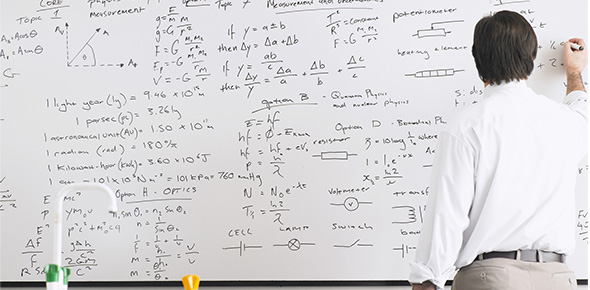# Do You Know About Balancing Equations?

15 Questions | Total Attempts: 68SettingsHere you will prove your ability to recognize parts of an equation, whether or not it is balanced and balance it.

• 1.
Cu + HNO3 Cu(NO3)2 + NO + H2O Balance this reaction. What are the coefficients for each reactant/product in the reaction?
• A.

3, 4, 6, 5

• B.

3, 8, 3, 2, 4

• C.

2, 4, 4, 7, 2

• D.

1, 5, 4, 7, 2

• E.

6,2 , 2,4

• F.

None of the above

• 2.
C3H8(g) + O2(g) ----> CO2(g) + H2O(g) Balance this reaction. What are the coefficients for each reactant/product in the reaction?
• A.

1,4, 3, 4

• B.

3, 5, 4, 1

• C.

3, 3, 4, 2

• D.

1,3, 5, 2

• E.

1, 5, 3 , 4

• 3.
Ca(OH)2 + HCl ---> CaCl2+ H2O Balance this reaction. What are the coefficients for each reactant/product in the reaction?
• A.

1, 2, 1, 2

• B.

2, 2, 1, 3

• C.

1, 3, 4, 1

• D.

3, 3, 4, 1

• E.

2, 3, 1, 2

• 4.
Cu + HNO3 Cu(NO3)2 + NO + H2O Which of the following is a reactant?
• A.

Cu(NO3)2

• B.

NO

• C.

H2O

• D.

HNO3

• E.

None of these

• 5.
Al + O2  -->  Al2O3 What are the coefficients that would balance this reaction?
• A.

2, 3, 3

• B.

3, 4, 4

• C.

4, 3, 2

• D.

2, 4, 3

• E.

1, 3 ,2

• 6.
What law says we need to have balanced chemical equations?
• A.

The law of constant attention

• B.

• C.

The second law of thermodynamics

• D.

The law of conservation of mass

• E.

The rule of law

• 7.
______ CH­2O            +             ______ HCl ---->  ______ CH4O             +            ______ Cl2
• A.

1 2 1 1

• B.

2 1 1 1

• C.

1 1 2 1

• D.

1 1 1 2

• 8.
___ CH4 + ___O2 --> ___CO2 + ___H2O
• A.

1 2 1 2

• B.

2 1 2 1

• C.

3 1 2 4

• D.

1 2 3 4

• E.

4 3 1 2

• 9.
____ NH3+ ____ H2SO4 à ____ (NH4)2SO4  Write the coeficients below with a comma and space separating each.
• 10.
____ Pb + ____ H3PO4 à ____ H2 + ____ Pb3(PO4)2   Write the coeficients below with a comma and space separating each.
• 11.
Which is the balanced equation for silver nitrate added to chlorine gas making silver chloride and nitrogen gas and oxygen gas
• A.

AgNO2 + Cl2 ---> AgCl2 + N + O2

• B.

2AgNO2 + Cl2 ---> 2AgCl + N2 + 2O2

• C.

2AgNO3 + Cl2 ---> 2AgCl + N2 + 3O2

• D.

AgNO3 + Cl2 ---> AgCl2 + N + O2

• 12.
Which is the balanced equation for hydrogen gas reacting with nitrogen gas producing ammonia?
• A.

N2 + H2 ---> N2H2

• B.

3H2 + N2 ---> 2NH3

• C.

2H2 + 3N2 ---> 2N6H4

• D.

2N2 + 3H2 ---> 2N2H3

• 13.
Which is the balanced equation for magnesium added to copper(I) chloride yielding copper and magnesium chloride?
• A.

Mg + 2CuCl ---> 2Cu + MgCl2

• B.

Mg + CuCl ---> Cu + MgCl

• C.

2Mg + 4CuCl ---> 4Cu + 2MgCl2

• D.

Mg + CuCl2 ---> Cu + MgCl2

• 14.
Look at this reaction and identify the products: Mg + CuCl ---> Cu + MgCl
• A.

CuCl and Mg

• B.

Cu and Mg

• C.

Cu and MgCl

• 15.
What do the subscripts in this molecule tell you? H2SO4
• A.

That there are 2 sulfur atoms and 4 oxygen atoms

• B.

That there are 2 hydrogen atoms and 4 oxygen atoms

• C.

That there are 8 molecules of H2SO4

• D.

That there are 2 hydrogen atoms and 4 sulfur atoms

Related TopicsBack to top